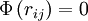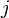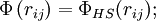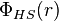# Widom-Rowlinson model$\Phi \left( r_{ij} \right) = 0$; if$i$ and$j$ are particles of the same species$\Phi \left( r_{ij} \right) = \Phi_{HS}(r_{ij});$ if$i$ and$j$ belong to different species,
where$\Phi_{HS}(r)$ is the hard sphere intermolecular pair potential.